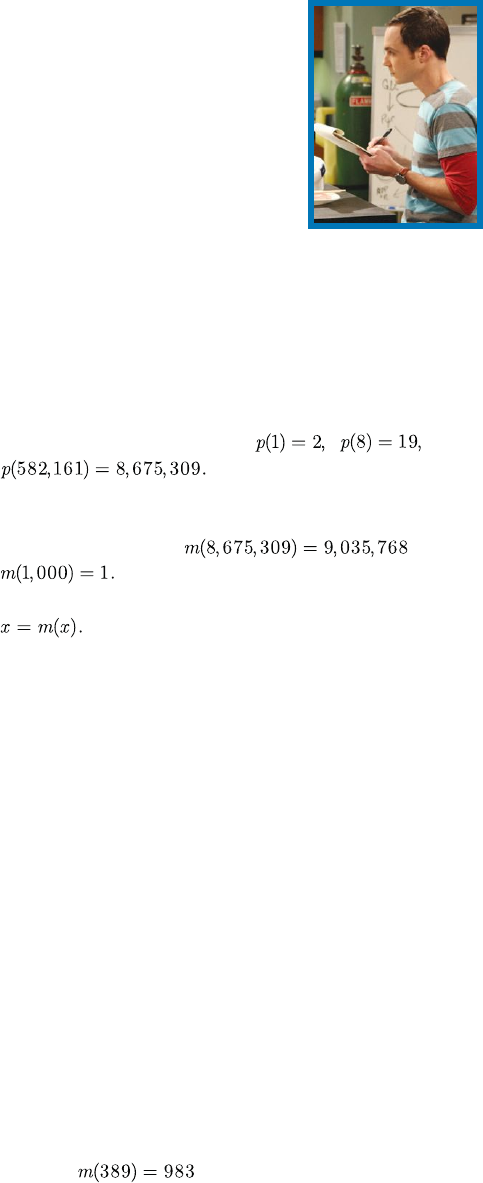Here's the video of the 73rd episode when Sheldon mentions his favo...
Except for 11, all palindromic primes have an odd number of digits,...
Here's a some Matlab code if you want to find prime mirrors: `...
A result of Rosser and Schoenfeld is that  \pi (x) \geq \fra...
In February 2019, Carl Pomerance and Chris Spicer were able to prov...12 November 2015 : : Math Horizons : : www.maa.org/mathhorizons
T
he Big Bang Theory is a CBS sitcom cre-
ated in 2007. It follows the escapades of ﬁve
friends: Sheldon Cooper, physicist; Leonard
aerospace engineer; Raj Koothrappali, as-
trophysicist; and Penny, a waitress and aspiring actress
who lives across the hall. In the 73rd episode of the
show, we hear the following conversation .
Sheldon: What is the best number? By the way,
Raj: Five million, three hundred eighteen thou-
sand, eight?
Sheldon: Wrong. The best number is 73. You’re
probably wondering why.
Leonard: No.
Howard: Uh-uh.
Raj: We’re good.
Sheldon: Seventy-three is the 21st prime number.
Its mirror, 37, is the 12th, and its mirror, 21, is the
product of multiplying, hang on to your hats, seven
and three. Eh? Eh? Did I lie?
Leonard: We get it. Seventy-three is the Chuck
Norris of numbers.
Sheldon: Chuck Norris wishes. In binary, 73 is a
palindrome, 1,001,001 which backwards is 1,001,001,
exactly the same. All Chuck Norris backwards gets
you is Sirron Kcuhc.
Sheldon is correct in each of his statements regarding
73. We will call a number that satisﬁes these properties
(which we deﬁne explicitly below) a Sheldon prime.
Implicit in Sheldon’s assertion that 73 is the best num-
ber is that 73 is the only Sheldon prime. We call this
the Sheldon conjecture.
Although we cannot prove the conjecture, we show
that a counterexample, if one exists, must be very large.
The Mirror Property
We begin by deﬁning some notation that will help
us simplify this problem. First, let p(n) be the nth
prime number. For example,
and
The mirror of a natural number x, which we denote
m(x), is the number obtained by reversing the order
of the digits of x. So
and
Some numbers satisfy the very special property that
These numbers are their own mirrors and
are called palindromic numbers. Palindromic primes,
such as 101 or 373, are rare and have been studied in
their own right . There are inﬁnitely many pal-
indromic numbers, but it is an open question as to
whether there are inﬁnitely many palindromic primes.
As of November 2014, the largest known palindromic
prime was 474,501 digits long .
With these deﬁnitions in hand, we are ready to state
the ﬁrst property of a Sheldon prime.
Deﬁnition. The nth prime number r satisﬁes the
mirror property if m(r) is the m(n)th prime number.
That is, m(p(n))=p(m(n)).
There are actually two statements in the above prop-
erty. The ﬁrst is that m(r) is a prime number (which is
not guaranteed). The second is that m(r) is the m(n)th
prime number (deﬁnitely not guaranteed). We will look
at each part of this property.
Palindromic primes satisfy the rst part of the
property, but they are not the only ones. For example,
389 and
are both prime. Although there
are several obvious conditions that would preclude a
prime from satisfying this property, such as starting
with a 2, 4, 5, 6, or 8, primes that satisfy this ﬁrst
half are common. Of the ﬁrst 10 million primes, 14.99
SHELDON
CONJECTURE
the
Jessie Byrnes, Chris Spicer, and Alyssa Turnquist
This content downloaded from 130.237.29.138 on Wed, 11 Nov 2015 15:02:03 UTC
All use subject to JSTOR Terms and Conditions